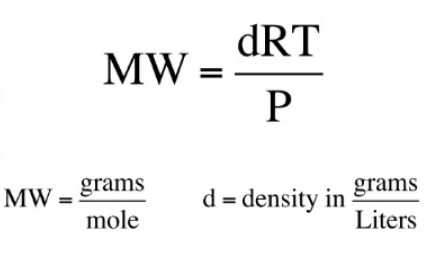# Problem: The density of a certain gaseous fluoride of phosphorus is 3.93 g/L at STP. Calculate the molar mass of this fluoride and determine its molecular formula.

###### FREE Expert Solution

Since we are given a value for density and we are looking for the molar mass we use a derivative of the ideal gas law given below:Aside from the given density (3.93 g/L), we should know that at STP, P = 1 atm and T = 273.15 K. We should also know that the ideal gas constant R = 0.08026 L•atm/mol•K.

79% (373 ratings)###### Problem Details

The density of a certain gaseous fluoride of phosphorus is 3.93 g/L at STP. Calculate the molar mass of this fluoride and determine its molecular formula.